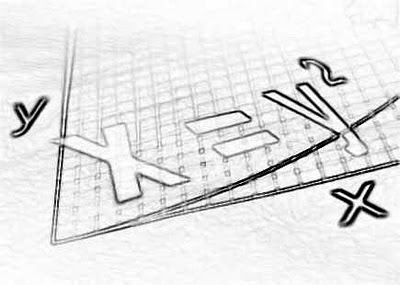11X + 23 = 4X – 5

That ↑ is called an equation.

My job is to find out the mystery number X.

First I get all the X’s on one side.

So I subtract 4X from both sides of the equation.

11X + 23 – 4X = 4X – 5 – 4X

7X + 23 = -5

Next I subtract 23 from both sides.

7X + 23 – 23 = -5 – 23

7X = -28

Now I need to turn the 7 into an invisible 1.

So we divide both sides by 7.

7X / 7 = -28 / 7

X = -4